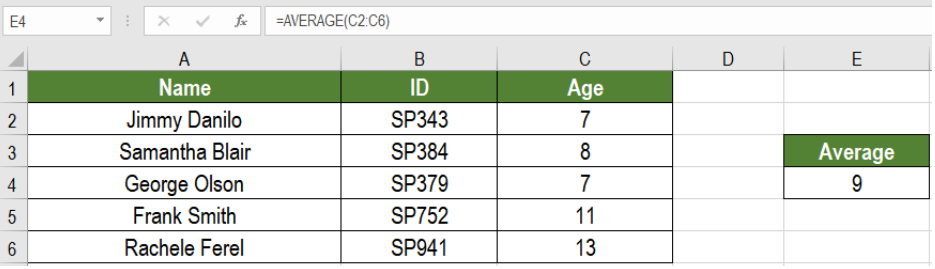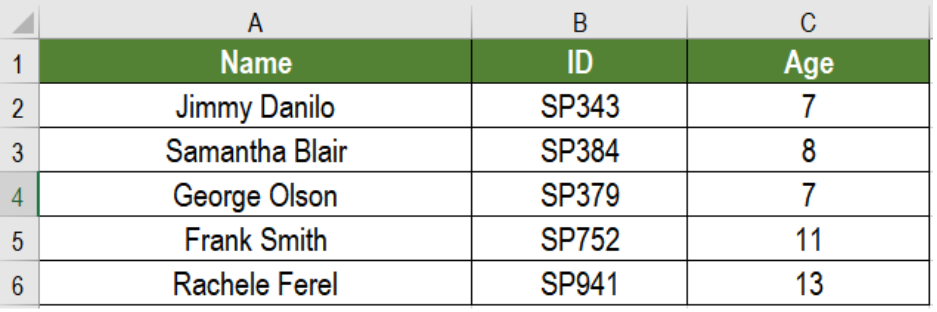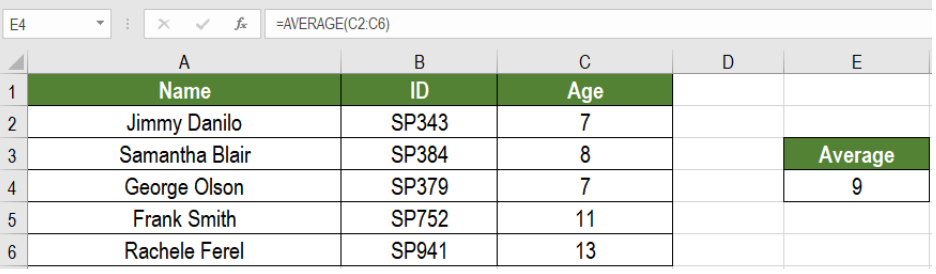Get instant live expert help with Excel or Google Sheets“My Excelchat expert helped me in less than 20 minutes, saving me what would have been 5 hours of work!”

#### Post your problem and you’ll get expert help in seconds.

Your message must be at least 40 characters
Our professional experts are available now. Your privacy is guaranteed.

# Average number

Excel offers some great features to work with numbers. Averaging numbers is one such task. The AVERAGE function can average numbers, taking up to 255 arguments individually. This allows it to work on large databases. In this tutorial, we will learn how to average numbers in Excel.Figure 1. Example of how to Average Numbers

## Formula

`=AVERAGE(range)`

## Process

This formula works in a very straightforward way. The AVERAGE function is automatic. It takes the only argument range. Here, we will provide a range of cells, Excel will average the numbers and return the result. If the cells are non-adjacent, we can provide individual cell references in the format `=AVERAGE (num1, [num2]...)`.

## Setting up Data

The following data set contains a student information data. Column A, B and C has the name, ID and ages of the students.Figure 2. The Sample Data Set

To get the average age in column E, we need to:

• Go to cell E4.
• Assign the formula `=AVERAGE(C2:C6) `to E4.
• Press Enter.

This will average the age and return the result 9 to E4.Figure 3. Applying the Formula

## Notes

1. Average does not count the blank cells and text included in the database.
2. It includes zero values.
3. Average can take numbers in any form, i.e. array or range from.

The AVERAGE function is a great way to quickly average numbers in Excel. It offers great flexibility to the users. Working with large databases are made very simple by AVERAGE.

Most of the time, the problem you will need to solve will be more complex than a simple application of a formula or function. If you want to save hours of research and frustration, try our live Excelchat service! Our Excel Experts are available 24/7 to answer any Excel question you may have. We guarantee a connection within 30 seconds and a customized solution within 20 minutes.

Are you still looking for help with the Average function? View our comprehensive round-up of Average function tutorials here.

### Did this post not answer your question? Get a solution from connecting with the expert.Another blog reader asked this question today on Excelchat:
Solution examplesOn the Grade Average worksheet, select G7 and type "Average." In cell G8, use a formula to generate the average of the contents in Cells B8:F8. Use the fill handle to populate your results through G10. The syntax for the Excel Average function is the following: AVERAGE(argument1, [argument2],...). Arguments can be numbers, named ranges, cell references that contain numbers, or arrays. Select Cell A12, and enter "Class Average." Use a formula to generate the average of IP Assignment 1 in cell B12. Use the fill across handle to populate your results of averaging the other assignments to F12
Solved by Z. A. in 40 minsI need help calculating average time elapsed. It is in dd:hh:mm:ss text format and so I can't calculate using =AVERAGEIF
Solved by O. E. in 11 minsI contacted you yesterdaya bout how to use the =AVERAGE function, and for real numbers it works fine, but I tried to get the expert to explain to me how to use the =AVERAGE function when the cells have formulas in them that generate '0' value, so the AVERAGE function would ignore these cells in the averaging method, but got no answer. So, I need to know how use the =AVERAGE function so that when there are formulas in the selected cells to average, that EXCEL would ignore those cells like they have real numbers in them, which seems to work fine. Please advise...
Solved by C. J. in 30 minsI need an IF formula where, IF there is a manual adjustment in a whole column of 100% values (say someone changes one to 90%) would say "Yes" or "No". Tried to solve this with =IF(AVERAGE(...)=100%, "No", "Yes") but it is kind of sloppy).
Solved by M. H. in 20 minsIF(AVERAGE(C6+E6+G6)>=5000,TRUE,FALSE) I am using AND function and it is not giving me the correct answer
Solved by G. J. in 24 mins## Subscribe to Excelchat.coAnother blog reader asked this question today on Excelchat: